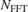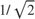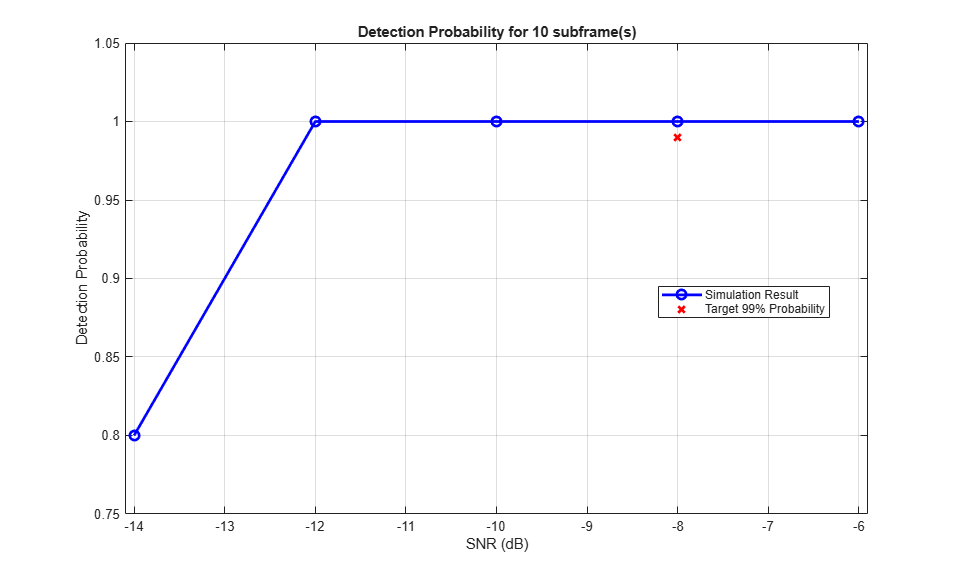# PRACH Detection Conformance Test

This example shows how the LTE Toolbox™ can be used to model a TS36.104 "PRACH Detection Requirements" conformance test. The probability of correct detection of the Physical Random Access Channel (PRACH) preamble is measured when the preamble signal is present.

### Introduction

The Random Access Channel (RACH) is an uplink transmission used by the User Equipment (UE) to initiate synchronization with the eNodeB. TS36.104, Section 8.3.4.1 [ 1 ] defines the probability of Physical Random Access Channel (PRACH) detection must be greater than or equal to 99% at an SNR of -8.0 dB. There are several detection error cases:

• Detecting an incorrect preamble

• Not detecting a preamble

• Detecting the correct preamble but with the wrong timing estimation

For channel propagation conditions ETU70, a timing estimation error occurs if the estimation error of the timing offset of the strongest path is larger than 2.08 us. The strongest path has a delay of 310 ns for ETU70.

In this example, a PRACH waveform is configured and passed through an appropriate channel. At the receiver PRACH detection is performed and the PRACH detection probability is calculated. The example is executed for the parameters defined in TS36.104, Section 8.4.1 [ 1 ] and TS36.141, Table A.6-1 [ 2 ]; i.e. normal mode, 2 receive antennas, ETU70 channel, normal cyclic prefix, burst format 0, SNR -8.0dB.

### Simulation Configuration

The test is carried out over 10 subframes at a number of SNRs. foffset is the frequency offset which will be modeled between transmitter and receiver and is specified for the test in TS36.104, Table 8.4.2.1-1 [ 1 ]. A large number of numSubframes should be used to produce meaningful results. SNRdB can be an array of values or a scalar.

numSubframes = 10; % Number of subframes to simulate at each SNR SNRdB = [-14.0 -12.0 -10.0 -8.0 -6.0]; % SNR points to simulate foffset = 270.0; % Frequency offset in Hertz 

### UE Configuration

User Equipment (UE) settings are specified in the structure ue.

ue.NULRB = 6; % 6 Resource Blocks ue.DuplexMode = 'FDD'; % Frequency Division Duplexing (FDD) ue.CyclicPrefixUL = 'Normal'; % Normal cyclic prefix length ue.NTxAnts = 1; % Number of transmission antennas 

### PRACH Configuration

The PRACH configuration is defined in the structure prach. This configuration is used to create a second structure info by calling ltePRACHInfo. This provides information about the PRACH generated given a specified configuration. Some of this information will be used later in the example.

prach.Format = 0; % PRACH format: TS36.104, Table 8.4.2.1-1 prach.SeqIdx = 22; % Logical sequence index: TS36.141, Table A.6-1 prach.CyclicShiftIdx = 1; % Cyclic shift index: TS36.141, Table A.6-1 prach.HighSpeed = 0; % Normal mode: TS36.104, Table 8.4.2.1-1 prach.FreqOffset = 0; % Default frequency location prach.PreambleIdx = 32; % Preamble index: TS36.141, Table A.6-1 info = ltePRACHInfo(ue, prach); % PRACH information 

### Propagation Channel Configuration

Configure the propagation channel model using a structure chcfg as per TS36.104, Table 8.4.2.1-1 [ 1 ].

chcfg.NRxAnts = 2; % Number of receive antenna chcfg.DelayProfile = 'ETU'; % Delay profile chcfg.DopplerFreq = 70.0; % Doppler frequency chcfg.MIMOCorrelation = 'Low'; % MIMO correlation chcfg.Seed = 1; % Channel seed chcfg.NTerms = 16; % Oscillators used in fading model chcfg.ModelType = 'GMEDS'; % Rayleigh fading model type chcfg.InitPhase = 'Random'; % Random initial phases chcfg.NormalizePathGains = 'On'; % Normalize delay profile power chcfg.NormalizeTxAnts = 'On'; % Normalize for transmit antennas chcfg.SamplingRate = info.SamplingRate; % Sampling rate 

### Loop for SNR Values

A loop is used to run the simulation for a set of SNR points, given by the vector SNRdB. The SNR vector configured here is a range of SNR points including a point at -8.0 dB, the SNR at which the test requirement for PRACH detection rate (99%) is to be achieved.

ltePRACH generates an output signal normalized to the same transmit power as for an uplink data transmissions within the LTE Toolbox. Therefore the same normalization must take place on the noise added to the PRACH. The noise added before SC-FDMA demodulation will be amplified by the IFFT. The amplification is the square root of the size of the IFFT (), thus to ensure the power of the noise added is normalized after demodulation, to achieve the desired SNR the desired noise power is divided by. In addition, as real and imaginary parts of the noise are created separately before being combined into complex additive white Gaussian noise, the noise amplitude must be scaled byso the generated noise power is 1.

At each SNR test point the probability detection is calculated on a subframe by subframe basis using the following steps:

• PRACH Transmission: A PRACH waveform is generated using ltePRACH with a specified timing offset. As per TS36.141, Section 8.4.1.4.2 [ 2 ] the preambles are sent with certain timing offsets defined in TS36.141, Figure 8.4.1.4.2-2 [ 2 ]. A timing offset base value is set to 50% of the number of cyclic shifts for PRACH generation. This offset is increased for each preamble, adding a step value of 0.1 us, until the end of the tested range, which is 0.9 us. This pattern then repeats.

• Noisy Channel Modeling: The waveform is passed through a fading channel and additive white Gaussian noise added. An additional 25 samples are added to the end of the waveform to cover the range of delays expected from the channel modeling (a combination of implementation delay and channel delay spread). This implementation delay is then removed the to ensure the implementation delay is interpreted as an actual timing offset in the preamble detector.

• Application of Frequency Offset: The frequency offset defined by the standard is also applied at this stage by performing a simple FM modulation of the received waveform.

• PRACH Detection: Perform PRACH detection using ltePRACHDetect for all cell preamble indices (0-63). The detected PRACH index and offset is returned and used to determine where a detection was successful according to the constraints discussed in the Introduction.

% Initialize the random number generator stream rng('default'); % Initialize variables storing probability of detection at each SNR pDetection = zeros(size(SNRdB)); for nSNR = 1:length(SNRdB) % Scale noise to ensure the desired SNR after SC-FDMA demodulation ulinfo = lteSCFDMAInfo(ue); SNR = 10^(SNRdB(nSNR)/20); N = 1/(SNR*sqrt(double(ulinfo.Nfft)))/sqrt(2.0); % Detected preamble count detectedCount = 0; % Loop for each subframe for nsf = 1:numSubframes % PRACH transmission ue.NSubframe = mod(nsf-1, 10); ue.NFrame = fix((nsf-1)/10); % Set PRACH timing offset in us as per TS36.141, Figure 8.4.1.4.2-2 prach.TimingOffset = info.BaseOffset + ue.NSubframe/10.0; % Generate transmit wave txwave = ltePRACH(ue, prach); % Channel modeling chcfg.InitTime = (nsf-1)/1000; [rxwave, fadinginfo] = lteFadingChannel(chcfg, ... [txwave; zeros(25, 1)]); % Add noise noise = N*complex(randn(size(rxwave)), randn(size(rxwave))); rxwave = rxwave + noise; % Remove the implementation delay of the channel modeling rxwave = rxwave((fadinginfo.ChannelFilterDelay + 1):end, :); % Apply frequency offset t = ((0:size(rxwave, 1)-1)/chcfg.SamplingRate).'; rxwave = rxwave .* repmat(exp(1i*2*pi*foffset*t), ... 1, size(rxwave, 2)); % PRACH detection for all cell preamble indices [detected, offsets] = ltePRACHDetect(ue, prach, rxwave, (0:63).'); % Test for preamble detection if (length(detected)==1) % Test for correct preamble detection if (detected==prach.PreambleIdx) % Calculate timing estimation error. The true offset is % PRACH offset plus channel delay trueOffset = prach.TimingOffset/1e6 + 310e-9; measuredOffset = offsets(1)/chcfg.SamplingRate; timingerror = abs(measuredOffset-trueOffset); % Test for acceptable timing error if (timingerror<=2.08e-6) detectedCount = detectedCount + 1; % Detected preamble else disp('Timing error'); end else disp('Detected incorrect preamble'); end else disp('Detected multiple or zero preambles'); end end % of subframe loop % Compute final detection probability for this SNR pDetection(nSNR) = detectedCount/numSubframes; end % of SNR loop 
Detected multiple or zero preambles Detected multiple or zero preambles 

### Analysis

At the end of the SNR loop, the calculated detection probabilities for each SNR value are plotted against the target probability.

hPRACHDetectionResults(SNRdB, numSubframes, pDetection);### Appendix

This example uses this helper function.

### Selected Bibliography

1. 3GPP TS 36.104 "Base Station (BS) radio transmission and reception"

2. 3GPP TS 36.141 "Base Station (BS) conformance testing"